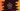# C++ program to print from a to z in different ways## C++ program to print from a to z:

In this post, we will learn how to print from a to z in C++ in different ways. We will use a for loop, while loop and do while loop to do the printing.

For printing the alphabet in lower case, we can run the loops using characters or using integers that holds the ASCII values of each character.

With this program, you will learn:

• How to use for loop in C++
• How to use while loop in C++
• How to use do-while loop in C++
• How to print content on console in C++

## Method 1: By using a for loop:

We can use a for loop to print a to z in C++. Below program uses a for loop:

``````#include <iostream>
using namespace std;

int main()
{
for (char c = 'a'; c <= 'z'; c++)
{
cout << c << ' ';
}
}``````

Here,

• The loop starts at c = ‘a’ and it runs until the value of c becomes ‘z’.
• On each iteration, it increment the value of c by 1. i.e. it moves to the next character.
• Inside the loop, we are printing the value of c. It also adds a space at the end of the character.

If you run this program, it will give the below output:

``a b c d e f g h i j k l m n o p q r s t u v w x y z``

## Method 2: C++ program to print from a to z separated by comma:

We can also add a comma between each character:

``````#include <iostream>
using namespace std;

int main()
{
for (char c = 'a'; c <= 'z'; c++)
{
cout << c;
c == 'z' ? cout << endl : cout << ',';
}
}``````

It is similar to the above program. The only difference is that it adds a comma to the right of a character if the character is not z, i.e. if it is not the last character to print. Else, it adds a comma after the character.

If you run this program, it will print the below line:

``a,b,c,d,e,f,g,h,i,j,k,l,m,n,o,p,q,r,s,t,u,v,w,x,y,z``

## Method 3: Using a while loop:

Let’s try to print a to z by using a while loop. It is similar to a for loop. The only difference is that it checks for one condition and it keeps the loop running until that condition is true.

``````#include <iostream>
using namespace std;

int main()
{
char c = 'a';

while (c <= 'z')
{
cout << c << ' ';
c++;
}
}``````

It will print:

``a b c d e f g h i j k l m n o p q r s t u v w x y z``

## Method 4: Using a do-while loop:

do-while is similar to while loop. We can use it in a similar way to print a to z:

``````#include <iostream>
using namespace std;

int main()
{
char c = 'a';

do
{
cout << c << ' ';
c++;
} while (c <= 'z');
}``````

It will print a similar output.

## Method 5: C++ program to print a to z using ASCII values:

Instead of using characters in a loop, we can also use the ASCII values of the characters. ASCII of a is 97 and z is 122. We can use ASCII to print these as like below:

``````#include <iostream>
using namespace std;

int main()
{
for (char c = 97; c <= 122; c++)
{
cout << c << ' ';
}
}``````

It will print:

``a b c d e f g h i j k l m n o p q r s t u v w x y z``